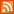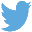Bucaro TecHelpHTTPS Encryption not required because no account numbers orpersonal information is ever requested or accepted by this siteCustom Search# Put Commas in Your Java Script Calculators

When humans deal with large numbers they write the digits in groups of three to make the numbers easier to read. Computers on the other hand don't need such aids and, in fact, commas in a number will confuse the heck out of basic program functions. That's why many online calculators require you to enter numbers without commas.

In this article you'll learn how to code calculator applications that will accept the entry of numbers with commas, and return results with commas. Actually, using a regular expression, it's very easy to remove commas from the users input value. An example of this is shown below.

```inputval = inputval.replace(/\,/g,'');
```

I generally avoid the use of regular expressions because they're too terse and complex for me to remember when I don't use them on a regular basis. When I use them, if later I need to fix a bug or update a piece of code, I need to re-learn how regular expressions work. But the regular expression above is very simple, it means replace all "," globally with nothing.

With the commons removed you can now pass the user's input to basic program functions. Now, if commas make it easier for humans to read numbers, it would be good to return a calculator's results with commons. On the Internet, I found a regular expression to do that, as shown below.

```s/(?<=\d)(?=(\d\d\d)+(?!\d))/,/g
```

Now you know what I mean by terse and complex. In fact if you search the Internet for regular expressions to put commas in numbers, you'll find most results asking why their regular expression doesn't work. On the Internet, I found a non-regular expression way to put commas in a number, as shown below.

```function CommaFormatted(amount)
{
var delimiter = ",";
amount = new String(amount);
var a = amount.split('.',2)
var d = a;
var i = parseInt(a);
if(isNaN(i)) { return ''; }
var minus = '';
if(i < 0) { minus = '-'; }
i = Math.abs(i);
var n = new String(i);
var a = [];
while(n.length > 3)
{
var nn = n.substr(n.length-3);
a.unshift(nn);
n = n.substr(0,n.length-3);
}
if(n.length > 0) { a.unshift(n); }
n = a.join(delimiter);
if(d.length < 1) { amount = n; }
else { amount = n + '.' + d; }
amount = minus + amount;
return amount;
}
```

This uses basic coding and is well formatted. But, I didn't actually try the code above because I thought I could come up with something even shorter and simpler. I came up with the code shown below.

```final = final.toFixed(2);
final = final.toString();
var farray = final.split(".");

var numGroups = (farray.length - farray.length % 3) /3;
var posLeft =  farray.length - (numGroups * 3);
var first = farray.substring(0,posLeft);
if(farray.length % 3 && farray.length > 3) first += ",";

for(var i = 0; i < numGroups; i++)
{
first += farray.substring(posLeft + (i * 3),posLeft + ((i + 1) * 3));
if(i < numGroups - 1) first += ",";
}

first = first + "." + farray;
```

Below, I explain how this code works, but first I'll mention two things. 1. In this code, I use the variable name "final" instead of "amount", as in this is the final value of a string of calculations and I'm now ready to insert the commas. 2. As you can see, I didn't really succeed in coming up with something shorter and simpler. But lets go ahead anyway.

 .menubtn { margin-top:10px; margin-left:50px; width:220px; opacity:1; border-style:solid; border-color:#009000; background-color:#66ff66; transition-property: opacity; transition-duration: 1s; transition-timing-function: ease-out; } .menubtn:hover { opacity:1; background-color:#e5ff23; } Menu - More Java Script ProgrammingRSS FeedFollow @Stephen Bucaro[Site User Agreement] [Privacy Policy] [Site map] [Search This Site] [Contact Form]
Copyright©2001-2021 Bucaro TecHelp 13771 N Fountain Hills Blvd Suite 114-248 Fountain Hills, AZ 85268